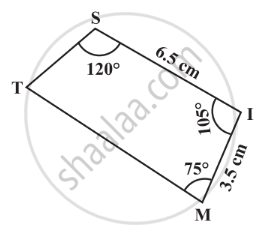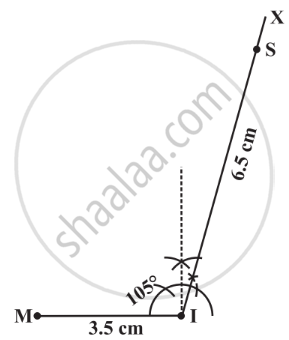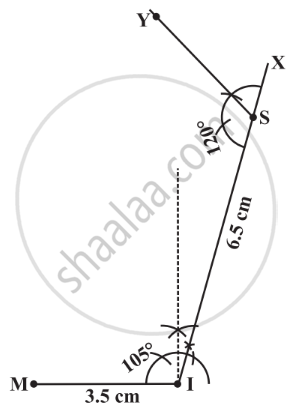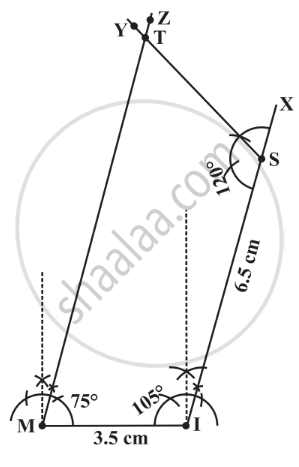Constructing a Quadrilateral When Two Adjacent Sides and Three Angles Are Known:

When two adjacent sides and three angles were known, we first drew a triangle with the available data and then tried to locate the fourth point.

1) Construct a quadrilateral MIST where MI = 3.5 cm, IS = 6.5 cm, ∠ M = 75°, ∠ I = 105° and ∠ S = 120°.

Solution:

Here is a rough sketch that would help us in deciding our steps of construction.Step 1: Draw a base MI with 3.5 cm. Make ∠ SIM = 105° at I.Step 2: Make ∠ ISY = 120° at S.Step 3: Make ∠IMZ = 75° at M. Mark that point as T. We get the required quadrilateral MIST.If you would like to contribute notes or other learning material, please submit them using the button below.